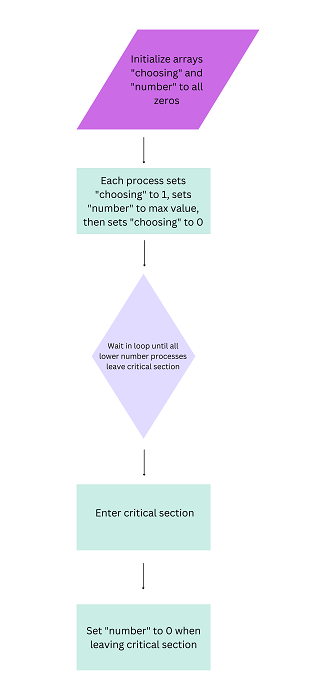# Lamport's Bakery Algorithm

A synchronization method called Lamport's Bakery method addresses the critical section issue in parallel computing systems. When more than one process needs to utilize a shared resource at once but only one process can do so, this is known as the critical section problem. To avoid conflicts and guarantee the accuracy of the system, the challenge is to make sure that each process uses the resource in a way that is mutually exclusive.

## Pseudo code for Lamport's Bakery Algorithm

Here the Pseudo code for Lamport’s Bakery Algorithm −

• Initialize an array, called choosing, of size N, where N is the total number of processes, to all zeros.

• Initialize an array, called the number, of size N, to all zeros.

• Each process i executes the following code when it wants to enter the critical section −

• Set choosing[i] = 1

• Set number[i] = max(number, number, ..., number[N-1]) + 1

• Set choosing[i] = 0

• For every other process j, repeat until (number[j] == 0) or (number[i], i) < (number[j], j): i. Wait

• Enter critical section

• Each process i executes the following code when it leaves the critical section −

• Set number[i] = 0## Code for Lamport's Bakery Algorithm

Here’s a code explaining Lamport’s Bakery Algorithm in action. We will be using C++ as the language of implementation in this example.

#include <iostream>
#include <atomic>

#define N 5
// total number of processes
using namespace std;

atomic<bool> entering[N] = {false};
// to keep track of which process is currently trying to enter critical section
atomic<int> number[N] = {0};
// to hold the ticket number for each process

void process(int i) {
while (true) {
// Step 1: Get ticket number
entering[i] = true;
int max_number = 0;
for (int j = 0; j < N; j++) {
if (number[j] > max_number) {
max_number = number[j];
}
}
number[i] = max_number + 1;
entering[i] = false;

// Step 2: Wait until it is this process's turn to enter the critical section
for (int j = 0; j < N; j++) {
while (entering[j]) {}
// wait until process j has finished choosing its ticket number
while ((number[j] != 0) && ((number[j] < number[i]) || ((number[j] == number[i]) && j < i))) {}
// busy wait until it is this process's turn to enter the critical section
}

// Step 3: Enter the critical section
cout << "Process " << i << " enters the critical section." << endl;
// perform critical section operations here

// Step 4: Exit the critical section
number[i] = 0;
cout << "Process " << i << " exits the critical section." << endl;
// perform remainder section operations here
}
}

int main() {
// create threads for each process
for (int i = 0; i < N; i++) {
}

for (int i = 0; i < N; i++) {
t[i].join();
}
return 0;
}


### Output

Process 0 enters the critical section.
Process 0 exits the critical section.
Process 1 enters the critical section.
Process 1 exits the critical section.
Process 2 enters the critical section.
Process 2 exits the critical section.
Process 3 enters the critical section.
Process 3 exits the critical section.
Process 0 enters the critical section.
Process 0 exits the critical section.
Process 1 enters the critical section.
Process 1 exits the critical section.
Process 4 enters the critical section.
Process 4Process  exits the critical section.2
.............


## Advantages for Lamport's Bakery Algorithm

Here are the advantages of Lamport's Bakery Algorithm listed below −

• distributing tokens in accordance with their designated values prevents starvation.

• Using a token-based strategy, it is simple and simple to comprehend and execute.

• efficient and doesn't need complicated data structures or interprocess interactions.

• without the need for specialized hardware or hardware assistance, it provides mutual exclusion.

• Widely applicable and adaptable, it can be used in many different situations to ensure fairness and reciprocal exclusion in concurrent computing.

• a useful tool for software engineers who work on distributed or parallel systems.

## Disadvantages of Lamport's Bakery Algorithm

• Busy waiting − The algorithm calls for busy waiting, which can result in inefficiencies and high CPU utilization, particularly when there are numerous processes or threads vying for access to the same shared resource.

• Starvation − Although the algorithm ensures justice, there are no safeguards against it. A process or thread may occasionally be repeatedly stopped, which prevents it from obtaining the token and gaining access to the resource.

• Overhead − The algorithm needs more memory and processing time to determine the sequence of tokens because it needs to store state information for each process or thread.

• Complexity − Because the algorithm must carefully handle race conditions and deadlocks, and possibly make use of synchronization mechanisms like mutexes or semaphores, its application can be difficult.

## Conclusion

A mutual exclusion algorithm called Lamport's Bakery Algorithm makes certain that various processes or threads can utilize a shared resource without interfering with one another. It is a straightforward algorithm that prevents starvation and ensures justice.

The algorithm works by allocating tokens to each process or thread that makes a request for access to the resource and then comparing the values of those tokens to determine the order in which they are given. The resource is first made available to the operation with the fewest tokens.

Updated on: 03-May-2023

667 Views